• 括号匹配问题描述：若表达式中包含三种括号：圆括号、方括号和花括号，它们可以相互嵌套。 算法思想：检验算法中可设置一个，每读入一个括号，若是左括号，则直接进栈，等待相匹配的同类右括号；若读入的是右括号...
栈结构具有后进先出的特点。括号匹配问题描述：若表达式中包含三种括号：圆括号、方括号和花括号，它们可以相互嵌套。

算法思想：检验算法中可设置一个栈，每读入一个括号，若是左括号，则直接进栈，等待相匹配的同类右括号；若读入的是右括号，且与当前栈顶左括号同类型，则二者匹配，将栈顶的左括号弹出，否则属于不合法情况。另外。如果输入序列已经读完，而栈中仍有等待匹配的左括号，或者读入一个右括号，而栈中已无等待匹配的同类型左括号，均属于不合法情况。当输入序列和栈同时变空的时候，说明所有括号完全匹配。

程序：括号匹配算法

#include<stdio.h>
#include<iostream>
#define FALSE 0
#define TRUE 1

using namespace std;
//链栈实现，采用链表作为存储结构来实现栈
typedef struct node{
char data;
struct node *next;

void bracketMatch(char *str);

bool match(char l,char r);

int main(){
char a;
cin.get(a,30); //有些类似getline。可以输入多个单词，中间空格隔开。
bracketMatch(a);
}

if(temp==NULL)   return(FALSE);//申请空间失败
temp->data=x;
temp->next=top->next;
top->next=temp;  //修改当前栈顶指针
return(TRUE);  //头插法

}
char *x;
temp=top->next;
if(temp==NULL)   return(FALSE);
top->next=temp->next;
*x=temp->data;
free(temp);
return(*x);
}
bool match(char l,char r){
bool judgematch=FALSE;
if((l='('&&r=')')||(l='['&&r=']')||(l='{'&&r='}'))
judgematch=TRUE;
return judgematch;
}

void bracketMatch(char *str){//str[]中为输入的字符串，利用堆栈技术检查该字符串中的括号是否匹配
int i;
char ch;
for(i=0;str[i]！='\0';i++){
switch(str[i]){
case '(':
case '[':
case '{':  //若为左括号则进栈
push(&s,str[i]);
break;
case ')':
case ']':
case '}':    //左括号时候
if(s->next==NULL){  //如果栈空
cout<<"右括号多余"<<endl;    return;}
else{
&ch=s->next->data;
if(match(ch,str[i]))
pop(&s,&ch);    //已匹配的左括号出栈
else{
cout<<"对应的左右括号不同类"<<endl; return;}
}
}
if(s->next=NULL)
cout<<"括号匹配"<<endl;
else
cout<<"左括号多余"<<endl;

}

}


在此还可以通过C++标准库（STL）中，实现了栈和队列，方便使用，并提供了若干方法。


展开全文stack
• ## C语言用栈实现括号匹配问题

千次阅读 多人点赞 2020-03-09 12:17:29
例如：{}[()]、{[()]}、()[]{}这种大中小括号成对出现（位置不限）则为括号匹配，反之则不匹配，如{()[ 接下来看一下实现方式 的定义以及相关操作 //的定义 typedef struct{ char elem[stack_size]; int top;...
例如：{}[()]、{[()]}、()[]{}这种大中小括号成对出现（位置不限）则为括号匹配，反之则不匹配，如{()[
接下来看一下实现方式

栈的定义以及相关操作
//栈的定义
typedef struct{
char elem[stack_size];
int top;
}seqStack;
//栈的初始化
void initStack(seqStack *s){
s->top=-1;
}
//判断栈空
int isEmpty(seqStack *s){
if(s->top==-1)
return 1;
else
return 0;
}
//入栈
int push(seqStack *s,char c){
if(s->top==stack_size-1)
return 0;
else{
s->top++;
s->elem[s->top]=c;
return 1;
}
}
//出栈
int pop(seqStack *s,char *x){
if(s->top==-1)
return 0;
else{
*x=s->elem[s->top];
s->top--;
return 1;
}

}

//获取栈顶元素
int gettop(seqStack *s,char *x){
if(!isEmpty(s)){
*x=s->elem[s->top];
return 1;
}
else
return 0;
}

括号处理
括号匹配的思想：依次从左至右检查字符串，若为左括号，则入栈，若遇右括号则获取栈顶元素，检查栈顶元素与当前元素是否匹配，若匹配，则栈顶元素出栈。反之，则不匹配，程序结束。
以此类推，直至检查完所有字符串。如果此时栈空则匹配，反之则不匹配。
//成对的左右括号的ASCII码相差1或者2，以此结论来判断左右括号是否成对出现
int match(char a,char b){
if(a+1==b||a+2==b)//成对的左右括号的ASCII码相差1或者2
return 1;
return 0;
}

int bracketMarch(char a[]){
seqStack s;
char ch;
int i;
initStack(&s);
for(i=0;a[i]!='\0';i++){
switch(a[i]){
case '{':
case '[':
case '(':
push(&s,a[i]);//遇到左括号则入栈
break;
case '}':
case ']':
case ')':
if(isEmpty(&s)){
return 0;
}
else{
gettop(&s,&ch);//遇到右括号获取栈顶元素
if(match(ch,a[i]))//检查是否匹配
pop(&s,&ch);//若匹配则栈顶元素出栈
else
return 0;
}
}
}
if(isEmpty(&s))//如果栈空，则括号是匹配的
return 1;
else//反之，则不匹配
return 0;
}

完整代码
#include<stdio.h>
#include<stdlib.h>
#include<string.h>
#define stack_size 100
//有关栈的操作

//栈的定义
typedef struct{
char elem[stack_size];
int top;
}seqStack;
//栈的初始化
void initStack(seqStack *s){
s->top=-1;
}
//判断栈空
int isEmpty(seqStack *s){
if(s->top==-1)
return 1;
else
return 0;
}
//入栈
int push(seqStack *s,char c){
if(s->top==stack_size-1)
return 0;
else{
s->top++;
s->elem[s->top]=c;
return 1;
}
}
//出栈
int pop(seqStack *s,char *x){
if(s->top==-1)
return 0;
else{
*x=s->elem[s->top];
s->top--;
return 1;
}

}

//获取栈顶元素
int gettop(seqStack *s,char *x){
if(!isEmpty(s)){
*x=s->elem[s->top];
return 1;
}
else
return 0;
}
int match(char a,char b){
if(a+1==b||a+2==b)//成对的左右括号的ASCII码相差1或者2
return 1;
return 0;
}
int bracketMarch(char a[]){
seqStack s;
char ch;
int i;
initStack(&s);
for(i=0;a[i]!='\0';i++){
switch(a[i]){
case '{':
case '[':
case '(':
push(&s,a[i]);//遇到左括号则入栈
break;
case '}':
case ']':
case ')':
if(isEmpty(&s)){
return 0;
}
else{
gettop(&s,&ch);//遇到右括号获取栈顶元素
if(match(ch,a[i]))//检查是否匹配
pop(&s,&ch);//若匹配则栈顶元素出栈
else
return 0;
}
}
}
if(isEmpty(&s))//如果栈空，则括号是匹配的
return 1;
else//反之，则不匹配
return 0;
}
int main(){
int n;
char a;
scanf("%d",&n);
while(n--){//你想检查几个字符串是否括号匹配？
scanf("%s",a);
if(bracketMarch(a))
printf("Yes\n");
else
printf("No\n");
}
}

ps:如有问题欢迎留言-


展开全文• 列表 元素在列表中是顺序存储的。 区分一下python和C语言储存列表（数组）的不同： C语言中①数组元素类型要...在python中的实现 写成了一个类。 class Stack: def __init__(self): self.stack = [] def pus
列表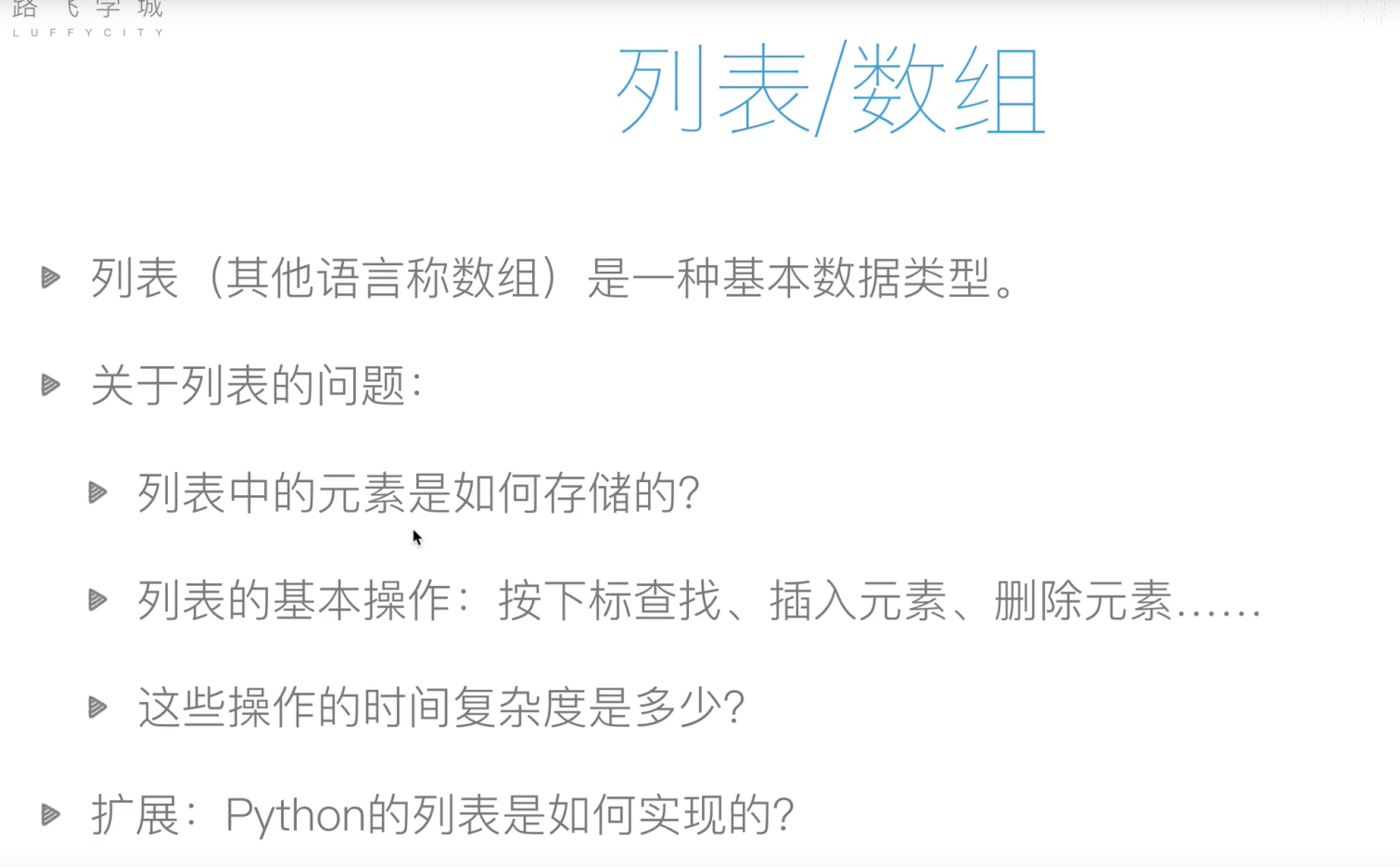元素在列表中是顺序存储的。
区分一下python和C语言储存列表（数组）的不同：
C语言中①数组元素类型要相同，②数组长度固定。
而在python中，地址的大小是固定的。把地址存储在列表中指向该列表中的数字。python的列表取数比在数组中取数字要多一步。
插入和删除在使用的过程中是O（n）的复杂度。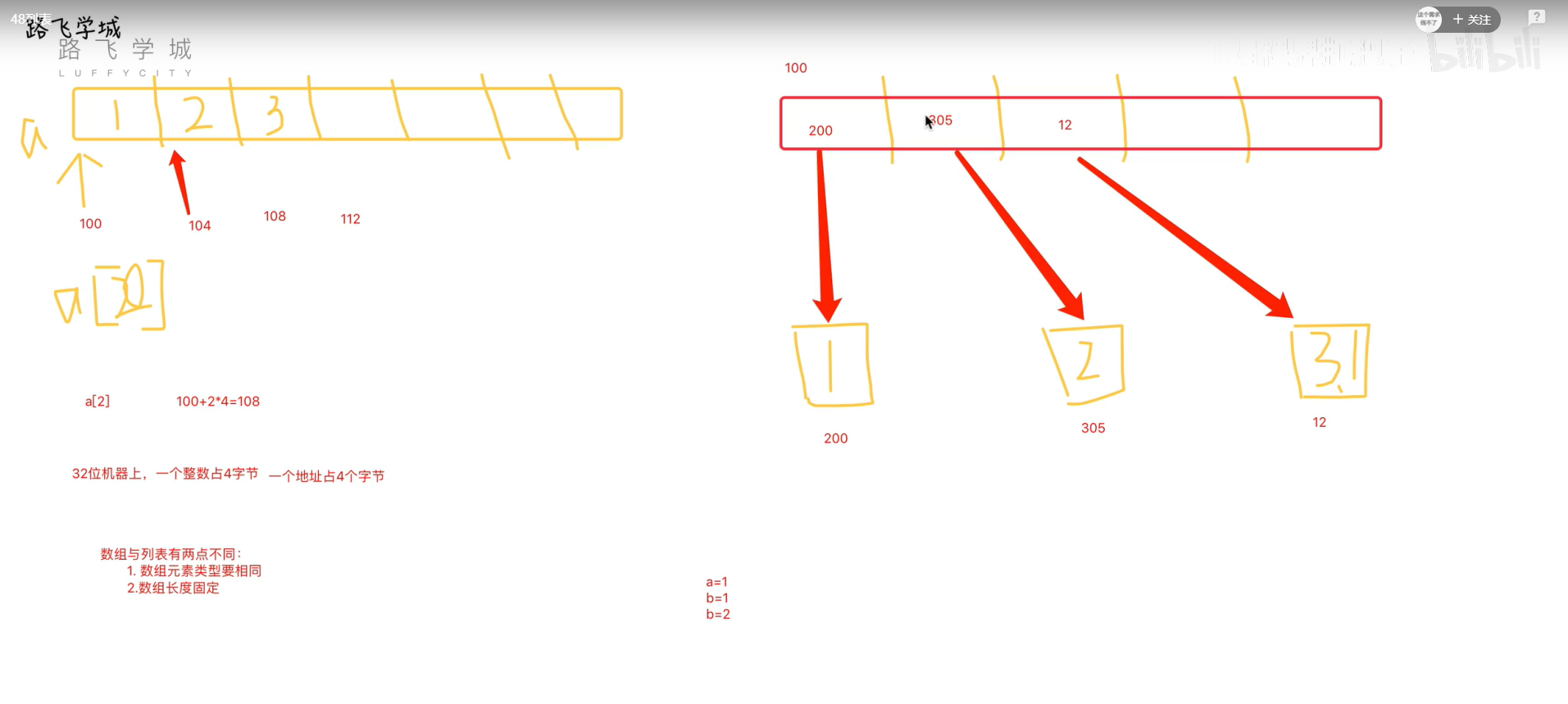栈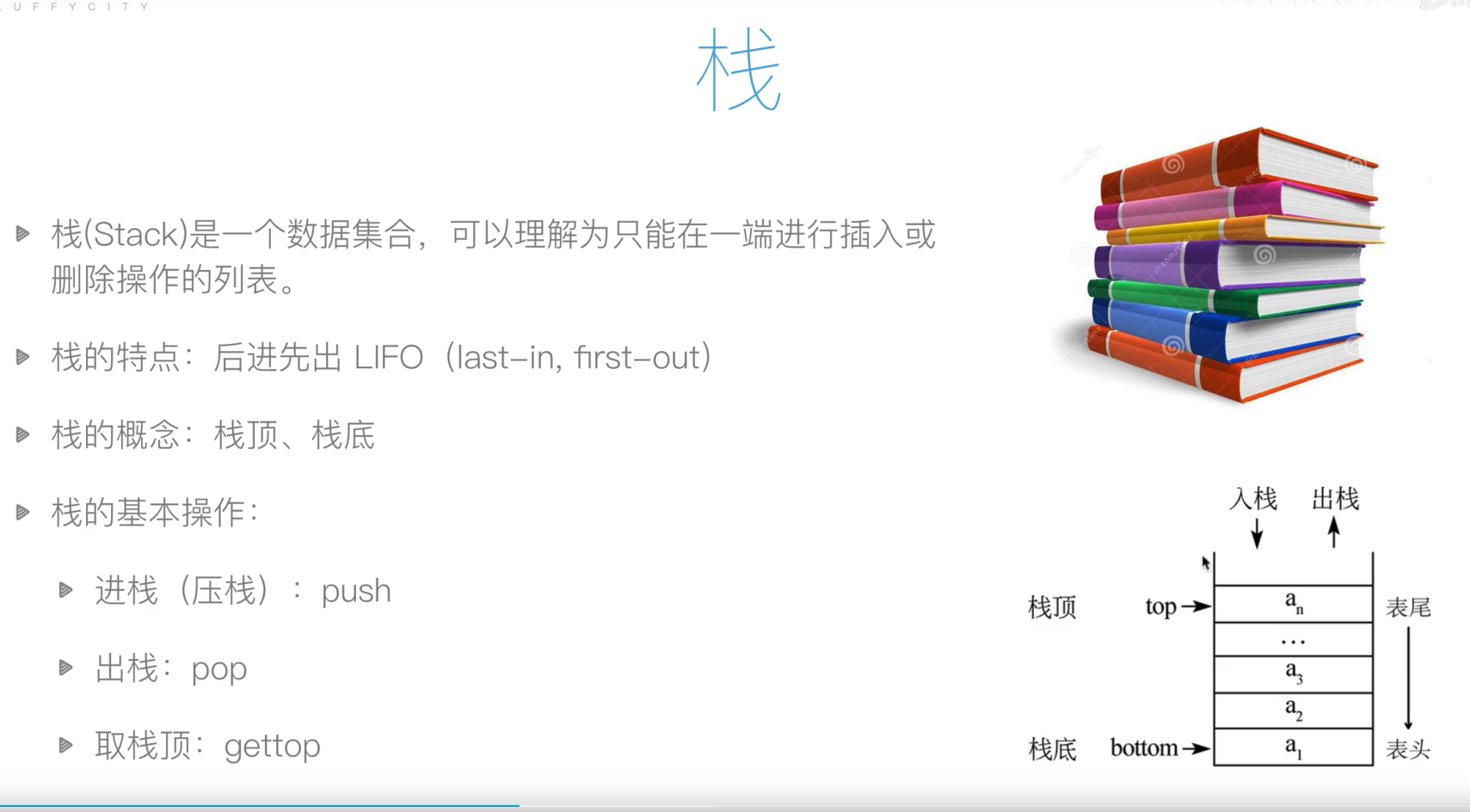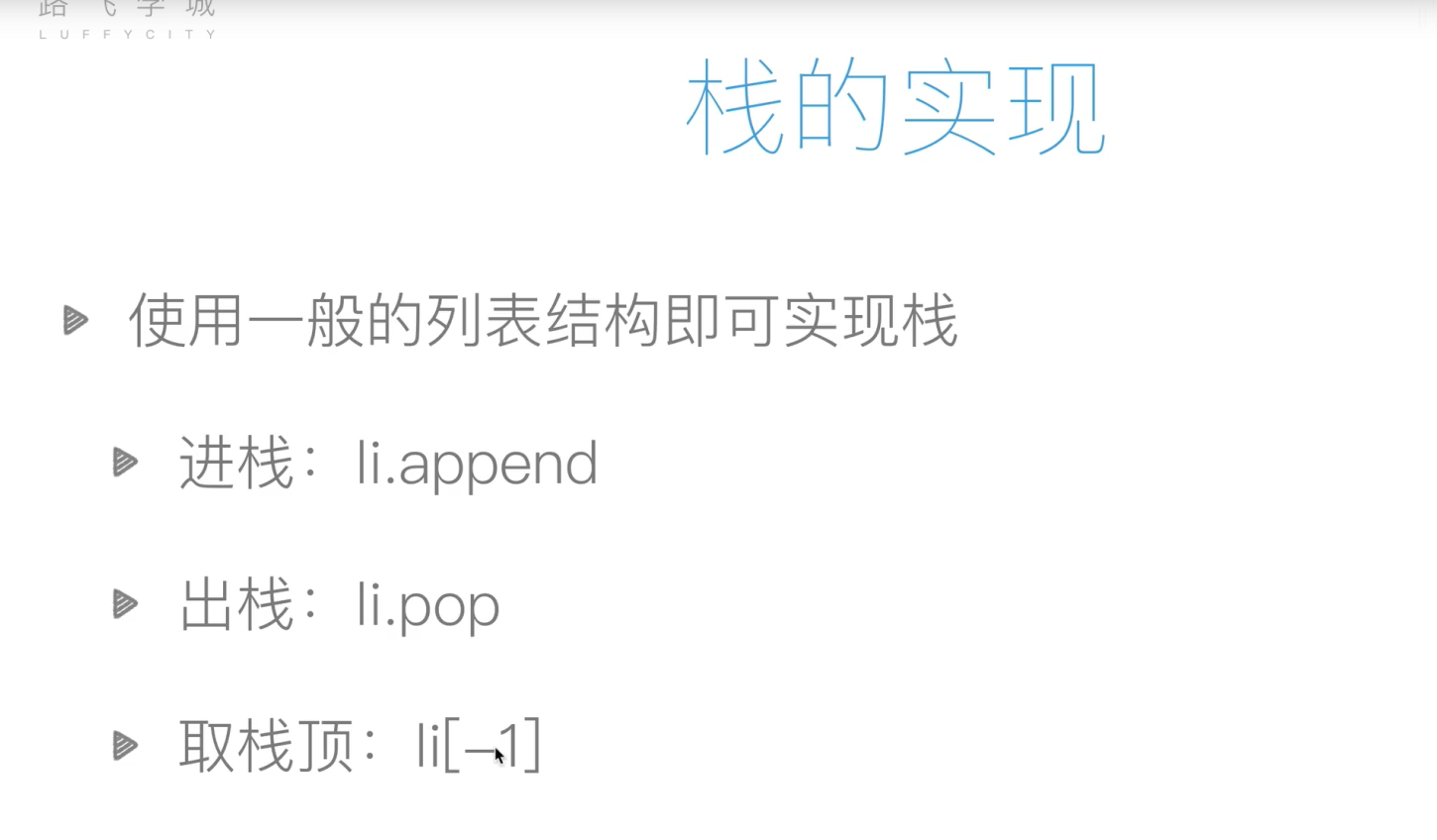栈在python中的实现
写成了一个类。
class Stack:

def __init__(self):
self.stack = []

def push(self, element):
self.stack.append(element)

def pop(self):
return self.stack.pop()

def get_top(self):
if len(self.stack) > 0:
return self.stack[-1]
else:
return None

stack = Stack()
stack.push(1)
stack.push(2)
stack.push(3)
print(stack.pop())
print(stack.get_top())

栈的应用：括号匹配问题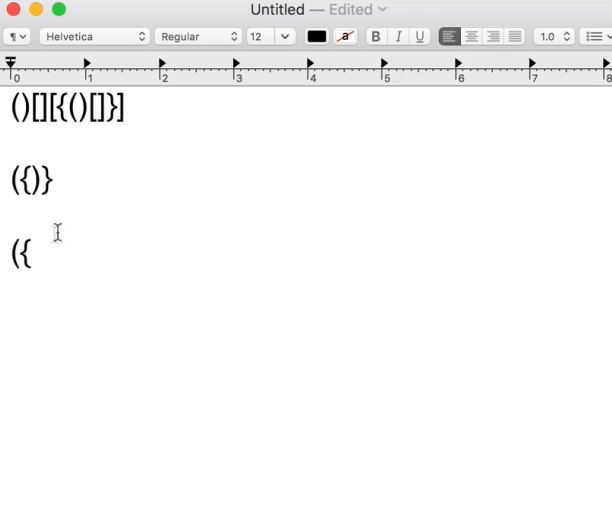左括号进栈，右括号出栈。当到最后一个右括号引起出栈行为，而且栈为空，就说明括号匹配。
续上面的栈生成类：
def brach_match(s):
match = {'}':'{', ']':'[', ')':'('}
stack = Stack()
for ch in s:
if ch in {'(', '[', '{'}:
stack.push(ch)
else:   # ch in {')', ']', '}'}
if stack.is_empty():
return False
elif stack.get_top() == match[ch]:
stack.pop()
else:   # stack.get_top() !== match[ch]
return False
if stack.is_empty():
return True
else:
return False

print(brach_match('{[]()}'))



展开全文列表 数据结构 python
• 请编写程序判断一个包含“（”和“）”的括号序列是否匹配。如匹配则输出Match；如不匹配，计算出使该序列变为匹配序列所需添加的最少括号数目（只允许在该序列开始和结尾处添加括号），并输出经添加最少括号后得到...
请编写程序判断一个包含“（”和“）”的括号序列是否匹配。如匹配则输出Match；如不匹配，计算出使该序列变为匹配序列所需添加的最少括号数目（只允许在该序列开始和结尾处添加括号），并输出经添加最少括号后得到的合法匹配序列。
输入格式:
输入为一个字符串，包含不超过100000个括号。
输出格式:
若输入的括号序列匹配，则输出Match。若不匹配，则输出分为2行，第1行为一个整数，表示将该序列变为匹配序列所需添加的最少括号数目，第2行为一个字符串，表示经添加最少括号后得到的合法匹配序列。
代码实现如下：
#include
using namespace std;
#define OK 1
#define ERROR 0
#define MAXSIZE 200000
typedef int Status;
typedef char SElemType;
typedef struct
{
SElemType *base;
SElemType *top;
int stacksize;
}SqStack;
Status InitStack(SqStack &S)
{
S.base=new SElemType[MAXSIZE];
if(!S.base) return ERROR;
S.top=S.base;
S.stacksize=MAXSIZE;
return OK;
}
Status Push(SqStack &S,SElemType e)
{
if(S.top-S.base==MAXSIZE) return ERROR;
*S.top++=e;
return OK;
}
Status Pop(SqStack &S,SElemType &e)
{
if(S.top==S.base) return ERROR;
e=*–S.top;
return OK;
}
SElemType GetTop(SqStack S)
{
if(S.top!=NULL)
return *(S.top-1);
}
Status StackEmpty(SqStack S)
{
if(S.top==S.base) return ERROR;
else return OK;
}
typedef struct
{
SElemType *elem;
int length;
}Sqlist;
Status InitList(Sqlist &L)
{
L.elem=new SElemType[MAXSIZE];
if(!L.elem) return ERROR;
L.length=0;
return OK;
}
Status ListInsert(Sqlist &L,int i,SElemType e)
{
int j;
if(i<1 || (i>L.length+1)) return ERROR;
if(L.length==MAXSIZE) return ERROR;
for(j=L.length-1;j>=i-1;j++)
L.elem[j+1]=L.elem[j];
L.elem[i-1]=e;
++L.length;
return OK;
}
void xianshi(Sqlist &L)
{
int i;
for(i=0;i<L.length;i++)
{
printf("%c",L.elem[i]);
}
printf("\n");
}
int main()
{
SqStack S;
InitStack(S);
Sqlist L;
InitList(L);
string ch;
cin>>ch;
int flag=1;
int n=0;
int b=0;
for(int i=0;i<ch.length();i++)
{
switch(ch[i])
{
case ‘(’:
Push(S,ch[i]);
ListInsert(L,i+1,ch[i]);
break;
case ‘)’:
if(StackEmpty(S) && GetTop(S)==’(’)
{
ListInsert(L,i+1,ch[i]);
Pop(S,ch[i]);
}
else
{
b++;
ListInsert(L,i+1,ch[i]);
flag=0;
n++;
}
break;
}
}
while(StackEmpty(S))
{
ListInsert(L,L.length+1,’)’);
n++;
S.top–;
}
if(flag)
cout<<“Match”;
else
{
cout<<n<<endl;
for(int i=0;i<b;i++)
cout<<"(";
xianshi(L);
}
return 0;
}
可能不是最优的算方法，但是能实现操作，欢迎大佬指正交流。


展开全文数据结构 堆栈
• 问题： 输入一系列的括号，判断格式是否正确这里直接java写好的实现较简单，不单独手写了，可以数组实现栈，也可以链表实现import java.util.Collection;import java.util.HashMap;import java.util...
• 链式栈实现括号匹配问题 思路 逐个字符录入（循环实现），如果录入到左括号就压栈 如果录入到右括号就弹栈 此时有两种情况： 1、栈空 则括号不匹配 2、栈不空 则括号可能匹配 对于第二种情况我们又分点 设置一个...
• 最近在复习数据结构，有关的一个问题括号匹配问题描述：输入一串括号，然后判断左右括号是不是匹配的。实验程序：/*brackets_match.c*/#include stdio.h> #define M 100 typedef struct Stack {  char...
• 问题： 输入一系列的括号，判断格式是否正确 这里直接用java写好的栈，栈的实现较简单，不单独手写栈了，可以用数组实现栈，也可以用链表实现 import java.util.... * 用栈解决括号匹配的类似问题 * */ publ.
• Stack的用例Parentheses，输入一串括号用栈判断其中的括号是否匹配，例如[()]{()}程序打印true，对于[](则打印false。思路： 遇到左括号入栈，遇到右括号时先检查栈是否为空，若空则返回false，若不空则弹出栈顶...java
• 1.问题 假设表达式中允许包含两种括号[ ]和( ),我们检验在表达式中是否是正确的格式，比如3+[3*(2-1)+1]是正确的格式，但是要是3+[(2+1])+1就不是正确的格式，那么我们如何进行检验呢 2.算法实现步骤 初始化一个...
• 如果是右括号，则判断是否为空，不为空则推出，为空就证明右括号没有匹配的项目，返回False 遍历结束之后判断是否为空，不为空则返回False，否则返回True python完整代码实现如下： class Stack(): def __init_...数据结构 stack
• （一） 问题描述 括号匹配问题是编译程序时经常遇到的问题，以检测语法是否有错。 （二） 基本要求 1. 顺序来检测括号是否匹配。 2. 令所给的式子中出现（）[ ]{ }这几种括号...null struct 存储 input 测试
• 在这里只做简单的一个括号匹配问题 其实思想都是一样的，若碰到左的，都入栈，右的，且原来的不为空，则拿出来进行匹配，成功了则继续往下做，否则，直接跳出循环。 在这里为了省略起见，了stl #include #...
• 问题描述： 输入一行括号 只包括’(’ ‘)’ ‘{’ ‘}’ ‘[’ ‘]’ 如果该字符串中所含的括号是配对的，则输出Yes,如果不配对则输出No。#include #include <stack>using namespace std;char change(char i) { ...
• 问题描述括号匹配问题，即给定一段文本text，括号是否有正确匹配的问题。括号由一个开括号如"("和一个闭括号")"组成，相互对应。这里只考虑三种括号，即"()"，"[]"，"{}"。此外括号括起的片段，也可能会嵌套。解决...
• 用栈实现括号匹配其实是一个很简单的问题，思路在代码注释里面写的很清楚了，只是接口设置的好像不太好。 如果在main里面设置的str不是动态分布的，在linux下就会出错，不知道windows会不会出问题。 kuohao.cpp #...
• 一个字符串中的括号的不匹配分为左括号多，右括号多或者次序问题在检测一个字符串中的字符如果是括号并且是左括号将它入栈，如果是右括号判断与栈顶元素是否匹配如果匹配将栈顶元素出栈如果不匹配返回false并输出...
• //队列实现栈 typedef struct { Queue q; } MyStack; MyStack* myStackCreate() { MyStack* ms = (MyStack*)malloc(sizeof(MyStack)); queueInit(&ms->q); return ms; } void myStackPush(My...
• 在结构化存储数据集是常常使用数组即...链表可以在O(1)的复杂度下处理删除，添加等问题，而且可以指针来动态分配存储空间，不会出现空间浪费的情况 例子： 编写一个Stack的用例，从输入中读取一个文本流并使用...数据结构
• 首先基于的基础上，实现括号匹配问题，要使用到的基本操作，入栈，获取栈顶元素，出栈等。 首先，先将左括号入栈，然后进行括号匹配，如果匹配成功，则让已经匹配成功的左括号出栈，继续进行匹配，直到外部的...数据结构...